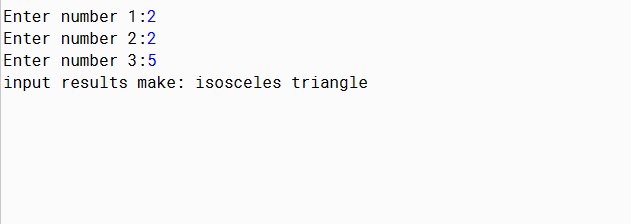Examples Problem Java Programming and Code

1.) Program determine type Triangle with Java

Flat Triangle is bounded by three sides and has three vertices. Triangle has some kind of like an isosceles triangle, equilateral triangle, the triangle is arbitrary, etc. By using java, we can create a program to determine the type of triangle side lengths just by entering the triangle side.

Examples of the coding like this:
``````
import java.util.Scanner;
public class DeterminingTriangle {
public static void main (String [] args) {
Scanner scanner = new Scanner (System.in);
int a, b, c;
System.out.print ("Enter number 1:");
a = scanner.nextInt ();
System.out.print ("Enter number 2:");
b = scanner.nextInt ();
System.out.print ("Enter number 3:");
c = scanner.nextInt ();
if(a==b&&b!=c&&a!=c||b==c&&b!=a&&a!=c||a==c&&b!=a&&b!=c) {
// if only two numbers have the same value then print an isosceles triangle
System.out.print ("input results make: isosceles triangle");
} else if (a == b || a == c || b == c) {
// if all three numbers are the same then print an equilateral triangle
System.out.print ("input results make: equilateral triangle");
} else
// if the three sides are not the same then print an arbitrary triangle
System.out.print ("the input results make: an arbitrary triangle");
}

}
``````coding result, in the example I put 2 fatherly produce the same number of isosceles triangles

2.) Determine the Largest Numbers Java program, Smallest, and the average

Next is a program that determines the largest number, smallest, and the average is determined by inputting three numbers. and by using the concept of branching.

Examples of the coding like this:

``````
import java.util.Scanner;
public class LargestSmallData {
public static void main (String [] args) {
System.out.println ("Specifies the Biggest, Smallest, and Average Numbers \n");
Scanner input = new Scanner (System.in);
double x;
double y;
double z;
double avg;
System.out.print ("Enter First Number (X):");
x = input.nextDouble ();
System.out.print ("Enter Second Number (Y):");
y = input.nextDouble ();
System.out.print ("Enter Third Number (Z):");
z = input.nextDouble ();
avg = (x + y + z) / 3;
System.out.println ("\n Results:");
System.out.println ("The average is:" + avg);
if (x == y && y == z) {System.out.println ("The Three Same Numbers are Large");
} else if (x> y && x> z) {System.out.println (x + "is the largest number");
} else if (y> z && y> x) {System.out.println (y + "is the largest number");
} else if (z> x && z> y) {System.out.println (z + "is the largest number");
} else if (x == y && x> z) {System.out.println (x + "is the largest number");
} else if (x == z && x> y) {System.out.println (x + "is the largest number");
} else {System.out.println (y + "is the largest number");
}
if (x == y && y == z) {System.out.println ("The Three Small Same Numbers");
} else if (x <y && x <z) {System.out.println (x + "is the smallest number");
} else if (y <z && y <x) {System.out.println (y + "is the smallest number");
} else if (z <x && z <y) {System.out.println (z + "is the smallest number");
} else if (x == y && x <z) {System.out.println (x + "is the smallest number");
} else if (x == z && x <y) {System.out.println (x + "is the smallest number");
} else {System.out.println (y + "is the smallest number");
}
}
}
``````coding result, in the example above shows the input of the largest, smallest and the average of the three input is entered

3.) For Counting Acquisition Bonus Program

Based java program serves to calculate the total price and bonus purchased
If the amount of price in the purchasing = 1500000 will earn bonus Iron
If the amount of price in the purchasing = 1000000 will get a bonus Umbrella
If the amount of price in the purchasing = 500000 then will get bonuses Ballpoint

Examples of the coding like this
``````
import java.util.Scanner;
public class Bonuses {
public static void main (String [] args) {
// TODO code application logic here
Scanner enter = new Scanner (System.in);
String Bonus = null;

System.out.print ("Enter Amount of Purchases =");
int A = enter.nextInt ();
int D1 = A * 500000;
System.out.print ("Buy Total Amount =" + A);
System.out.print ("\n");
int result = ((D1));
System.out.print ("-------------------------- \n");
System.out.print ("Total price =" + result);
System.out.print ("\n");

if (result>= 1500000) {Bonus = "CONGRATS YOU GET AN IRON BONUS";}
else if (result>= 1000000) {Bonus = "CONGRATS YOU GET UMBRELLA BONUS";}
else if (result>= 500000) {Bonus = "CONGRATS YOU GET BONUS BALLPOINT";}
else {Bonus = "SEE YOU AGAIN TO COME BACK";}
System.out.println ("" + Bonus);
}
}

``````coding results, in my example input 2 so that the total price to 1000000 and I get bonuses umbrella

Berlangganan update artikel terbaru via email: